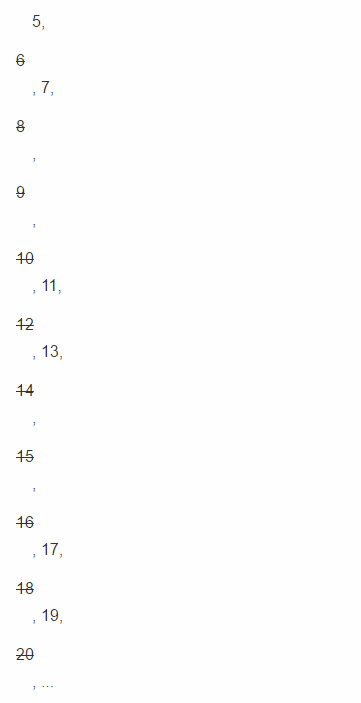# Python基础之常见内建函数发表于

### map()

`map()` 函数接受两个参数，一个是函数，一个是可迭代对象（Iterable）, `map` 将传入的函数依次作用到可迭代对象的每一个元素，并把结果作为迭代器(Iterator)返回。

>>> def f(x):

...     return x * x

...

L = []

for n in [1, 2, 3, 4, 5, 6, 7, 8, 9]:

L.append(f(n))

print(L)

>>> r = map(f, [1, 2, 3, 4, 5, 6, 7, 8, 9])

>>> list(r)

[1, 4, 9, 16, 25, 36, 49, 64, 81]

>>> list(map(str, [1, 2, 3, 4, 5, 6, 7, 8, 9]))

['1', '2', '3', '4', '5', '6', '7', '8', '9']

def normalize(name):

return name.capitalize()

l2=list(map(normalize,l1))

print(l2)

### reduce()

`reduce()` 函数也是接受两个参数，一个是函数，一个是可迭代对象， `reduce` 将传入的函数作用到可迭代对象的每个元素的结果做累计计算。然后将最终结果返回。

>>> from functools import reduce

>>> def fn(x, y):

...           return x * 10 + y

...

>>> reduce(fn, [1, 2, 3, 4, 5])

12345

from functools import reduce

def pro (x,y):

return x * y

def prod(L):

return reduce(pro,L)

print(prod([1,3,5,7]))

`map()` 和 `reduce()` 综合练习：编写 `str2float` 函数，把字符串 `'123.456'` 转换成浮点型 `123.456`

CHAR_TO_FLOAT = {

'0': 0,'1': 1,'2': 2,'3': 3,'4': 4,'5': 5,'6': 6,'7': 7,'8': 8,'9': 9, '.': -1

}

def str2float(s):

nums = map(lambda ch:CHAR_TO_FLOAT[ch],s)

point = 0

def to_float(f,n):

nonlocal point

if n==-1:

point =1

return f

if point ==0:

return  f*10+n

else:

point =point *10

return  f + n/point

return  reduce(to_float,nums,0)#第三个参数0是初始值，对应to_float中f

### filter()

`filter()` 函数用于过滤序列， `filter()` 也接受一个函数和一个序列，`filter()` 把传入的函数依次作用于每个元素，然后根据返回值是 `True` 还是 `False` 决定保留还是丢弃该元素。

def is_odd(n):

return n % 2 == 1

list(filter(is_odd, [1, 2, 4, 5, 6, 9, 10, 15]))

# 结果: [1, 5, 9, 15]

2, 3, 4, 5, 6, 7, 8, 9, 10, 11, 12, 13, 14, 15, 16, 17, 18, 19, 20, ...def _odd_iter():

n = 1

while True:

n = n + 2

yield n

#这是一个生成器，并且是一个无线序列

def _not_divisible(n):

return lambda x: x % n > 0

def primes():

yield 2

it = _odd_iter() # 初始序列

while True:

n = next(it) # 返回序列的第一个数

yield n

it = filter(_not_divisible(n), it) # 构造新序列

for n in primes():

if n < 100:

print(n)

else:

break

### sorted()

python内置的 `sorted()` 函数可以对list进行排序：

>>> sorted([36, 5, -12, 9, -21])

[-21, -12, 5, 9, 36]

`sorted()` 函数也是一个高阶函数，还可以接受一个 `key` 函数来实现自定义排序：

>>> sorted([36, 5, -12, 9, -21], key=abs)

[5, 9, -12, -21, 36]

`key` 指定的函数将作用于list的每一个元素上，并根据 `key` 函数返回的结果进行排序.

>>> sorted(['bob', 'about', 'Zoo', 'Credit'], key=str.lower)

>>> sorted(['bob', 'about', 'Zoo', 'Credit'], key=str.lower, reverse=True)

L = [('Bob', 75), ('Adam', 92), ('Bart', 66), ('Lisa', 88)]

def by_score(t):

for i in t:

return t

L2=sorted(L,key= by_score)

print(L2)

L2=sorted(L,key=lambda t:t) print(L2)